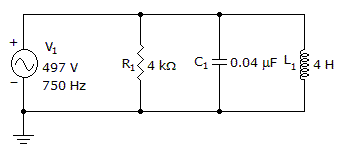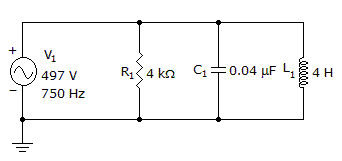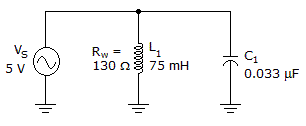# Electronics - RLC Circuits and Resonance

### Exercise :: RLC Circuits and Resonance - General Questions

41.

What is the power factor?A. 0.279 B. 0.479 C. 0.679 D. 0.879

Answer: Option D

Explanation:

No answer description available for this question. Let us discuss.

42.

What is the phase angle?A. 61.4º B. 28.5º C. -28.5º D. -61.4º

Answer: Option C

Explanation:

No answer description available for this question. Let us discuss.

43.

What would be the power factor for an RLC circuit that acts inductively?

 A. +90 degrees leading B. one C. zero D. –90 degrees lagging

Answer: Option C

Explanation:

No answer description available for this question. Let us discuss.

44.

Voltage lags current in an RLC circuit when it acts:

 A. capacitively B. resistively C. inductively D. resonantly

Answer: Option A

Explanation:

No answer description available for this question. Let us discuss.

45.What is the bandwidth of the circuit in the given circuit?

 A. 11.6 Hz B. 275.9 Hz C. 1.5 kHz D. 3.2 kHz

Answer: Option B

Explanation:

No answer description available for this question. Let us discuss.

#### Current Affairs 2021

Interview Questions and Answers